Home > CC4 > Chapter 11 > Lesson 11.1.2 > Problem11-22

11-22.
1.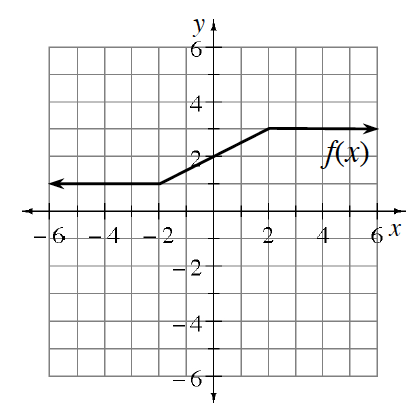Given the graph of f(x) at right, graph each transformation listed below. Homework Help ✎

1. f(x) − 3

2. f(x) + 2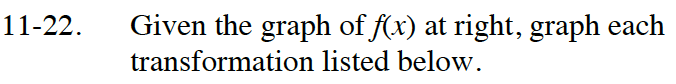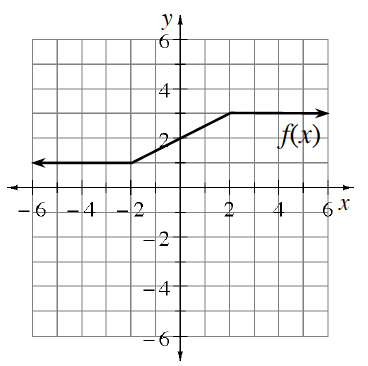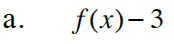Subtract 3 from each y-value on the graph. How will this shift the graph?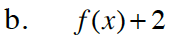Refer to part (a).